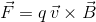# AP Physics C Electricity : Calculating Magnetic Fields and Forces

## Example Questions

### Example Question #1 : Calculating Magnetic Fields And Forces

An infinitely long wire has a current of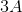running through it. Calculate the magnetic field at a distance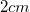away from the wire.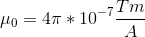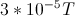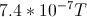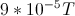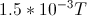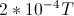Explanation:

For infinitely long wires, the formula for the magnetic field is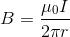, where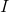is the current andis the distance from the wire.

The magnetic field is calculated using our given values.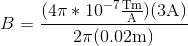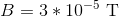### Example Question #2 : Calculating Magnetic Fields And Forces

A solenoid is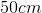long and it is made up of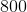turns of wire. How much current must run through the solenoid to generate a magnetic field of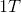inside of the solenoid?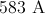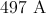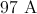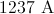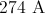Explanation:

The formula for the magnetic field inside the solenoid is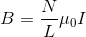, whereis the number of turns of wire,is the length of the solenoid, andis the current.

We want to find the current so we solve for.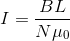Plug in the values.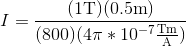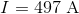### Example Question #3 : Calculating Magnetic Fields And Forces

Two parallel wires a distanceapart each carry a current, and repel each other with a forceper unit length. If the current in each wire is doubled to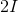, and the distance between them is halved to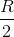, by what factor does the force per unit length change?Explanation:

Relevant equations: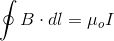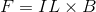Step 1: Find the original and new magnetic fields created by wire 1 at wire 2, using Ampere's law with an Amperian loop of radiusor, respectively.

Original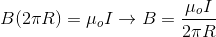New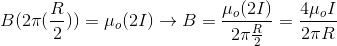Since the wires are parallel to each other and wire 1's field is directed circularly around it, in each case wire 1's field is perpendicular to wire 2.

Step 2: Find the original and new magnetic forces per unit length on wire 2, due to the field created by wire 1.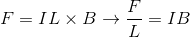Original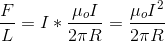New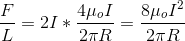So, the new force per unit length is 8 times greater than the original.

### Example Question #4 : Calculating Magnetic Fields And Forces

A region of uniform magnetic field,, is represented by the grey area of the box in the diagram. The magnetic field is oriented into the page.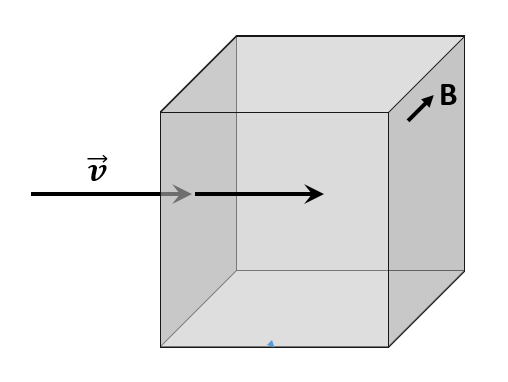A stream of protons moving at velocity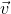is directed into the region of the magnetic field, as shown. Identify the correct path of the stream of protons after they enter the region of magnetic field.

A semi-circular path oriented vertically upward

A semi-circular path oriented vertically downward

A semi-circular path oriented horizontally into the page

A semi-circular path oriented horizontally out of the page